# Visualization in Python

`import pandas as pdimport numpy as npimport matplotlib.pyplot as pltimport seaborn as sns`
`#loading data with seaborndf_titanic = sns.load_dataset('titanic')df_iris = sns.load_dataset('iris')df_flights = sns.load_dataset('flights')`
• Univariate Plots
• Bivariate Plots
• Multivariate Plots
• Numerical Variables against Categorical Variables

# Univariate Plots

`plt.figure(figsize=(16,8))sns.distplot(df_titanic['fare'])plt.title('Distribution of Fare in titanic')plt.show()`
`#countplotplt.figure(figsize=(12,6))sns.countplot(df_titanic['sex'])plt.show()`

# Bivariate Plots

`plt.bar(df_flights['month'],df_flights['passengers'] )`
`sns.scatterplot(x='sepal_length', y='sepal_width', data=df_iris)plt.title('scaterplot in seaborn')`
`plt.scatter(df_iris['sepal_length'],df_iris['sepal_width'])plt.title('Scatter plot in matplotlib')plt.show()`
`sns.jointplot(x=df_iris['sepal_length'], y=df_iris['sepal_width'], kind='hex')`

# Multivariate Plots

`sns.scatterplot(x='sepal_length', y='sepal_width', hue='species', data =df_iris)plt.title('Scatterplt with hue in Seaborn')`
`sns.barplot(x='sex', y='fare', data= df_tiitanic, hue='class')`

# Numerical Variables against Categorical Variables

`sns.boxplot(x='sex', y='age', data = df_titanic)plt.show()`
`sns.boxplot(x='species', y='sepal_length', data = df_iris)`
`sns.violinplot(x='month', y='passengers', data=df_flights)`
`sns.swarmplot(y=df_iris['petal_length'], x=df_iris['species'])`
`sns.pairplot(df_iris, hue='species', diag_kind='hist')`
`g = pd.plotting.scatter_matrix(df_iris, figsize=(10,10), marker = '*', hist_kwds = {'bins': 10}, s = 60, alpha = 0.8)plt.show()`

--

--

--

## More from Akshar Rastogi

Love podcasts or audiobooks? Learn on the go with our new app.

## Connecting to international institutions data: the SDMX network and the pandasdmx library.## How I won my sixth bronze medal by entering Kaggle’s 2021 survey EDA competition## 6 Simple (But Important) Candlestick Patterns To Remember in Trading## Data visualization: integrity and authenticity for greater influence## Digital Analytics MiniDegree — Week 8 of 12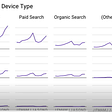## Finding Abandoned Places with SQL## A case study with an Airbnb competitor: using feature engineering to best predict property price…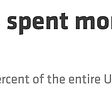## Rater Agreement in SAS using the Weighted Kappa and Intra-Cluster Correlation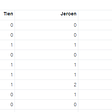## Show Geographical Data on Fascinating Maps Using Python## Collecting Historical Weather Data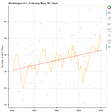## Data Visualization in Python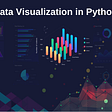## GIS project with GeoPandas